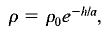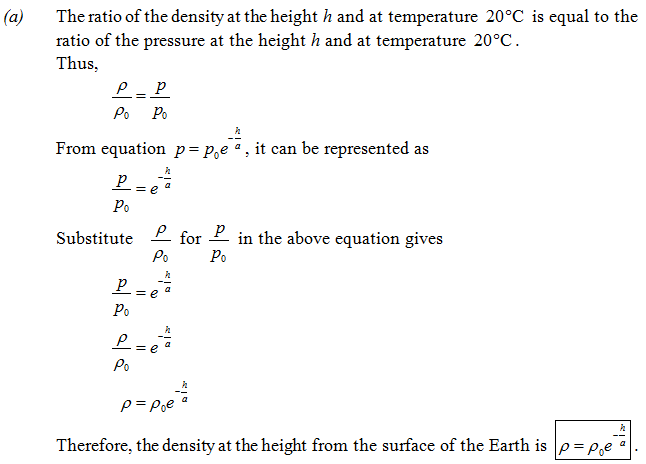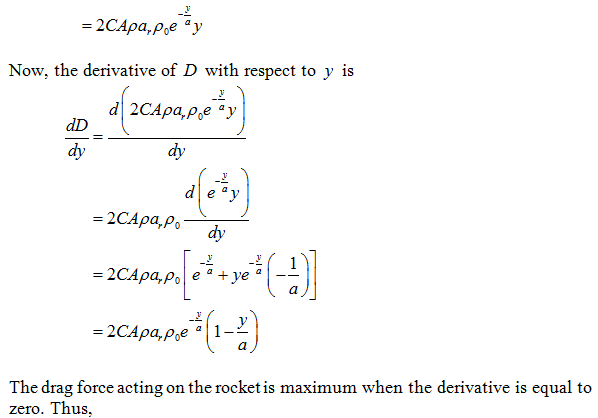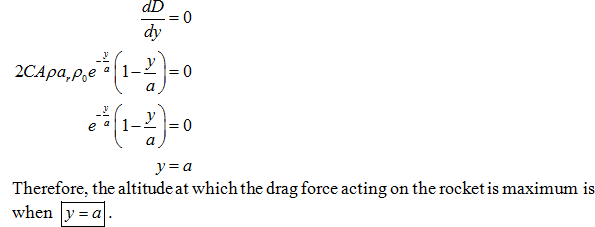×#### Thank you for registering.

One of our academic counsellors will contact you within 1 working day.

Click to Chat

1800-1023-196

+91-120-4616500

CART 0

• 0

MY CART (5)

Use Coupon: CART20 and get 20% off on all online Study Material

ITEM
DETAILS
MRP
DISCOUNT
FINAL PRICE
Total Price: Rs.

There are no items in this cart.
Continue Shopping
```
(a) Show that Eq. 15-13, the variation of pressure with altitude in the atmosphere (temperature assumed to be uniform), can be written in terms of density p as where P o is the density at the ground (11 = 0). (b) Assume that the drag force D due to the air on an object moving at speed v is given by D = CApv 2 where C is a constant, A is the frontal cross-sectional area of the object, and p is the local air density. Find the altitude at which the drag force on a rocket is a maximum if the rocket is launched vertically and moves with constant upward acceleration a.
(a) Show that Eq. 15-13, the variation of pressure with altitude in the atmosphere (temperature assumed to be uniform), can be written in terms of density p aswhere Po is the density at the ground (11 = 0). (b) Assume that the drag force D due to the air on an object moving at speed v is given by D = CApv2 where C is a constant, A is the frontal cross-sectional area of the object, and p is the local air density. Find the altitude at which the drag force on a rocket is a maximum if the rocket is launched vertically and moves with constant upward acceleration a.

```
5 years ago

``````
5 years ago
Think You Can Provide A Better Answer ?

## Other Related Questions on Mechanics

View all Questions »### Course Features

• 101 Video Lectures
• Revision Notes
• Previous Year Papers
• Mind Map
• Study Planner
• NCERT Solutions
• Discussion Forum
• Test paper with Video Solution### Course Features

• 110 Video Lectures
• Revision Notes
• Test paper with Video Solution
• Mind Map
• Study Planner
• NCERT Solutions
• Discussion Forum
• Previous Year Exam Questions